Back to Contents

# Looking Things Up

Many programs make use of a structure known as a dictionary. A real dictionary is used for associating definitions with words; we use “dictionary” more generally to mean associating some unique keys (like words) with values (like definitions). For example, we might like to store the following information about the number of people living in each house in a road: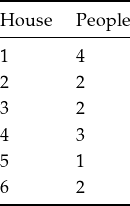The house number is the key, the number of people living in the house is the value. The order of keys is unimportant – we just need to be able to associate each key with one (and only one) value. It would be very inconvenient to store two lists, one of house numbers and one of people. For one thing, we would have way of guaranteeing the two lists were of equal length. What we would like is a way of representing pairs like (1, 4) and then having a single list of those. To make a pair in OCaml, just write it with parentheses and a comma: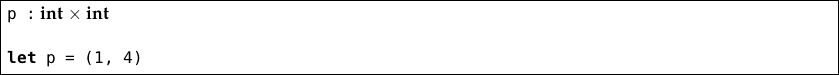It has the type int × int, which we pronounce as “int cross int”. When printed on the screen, `*` is used instead of × just as with multiplication. The two parts of the pair need not have the same type: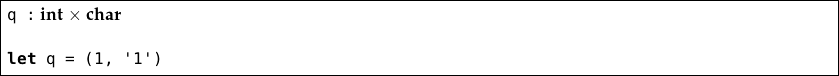We can write simple functions to extract the first and second element using pattern matching: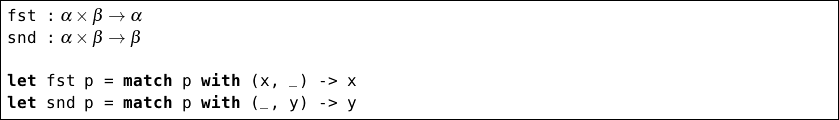In fact, since pairs can only take one form (unlike lists, which have two forms: empty or consisting of a head and a tail), OCaml lets us use the pattern directly in place of the argument: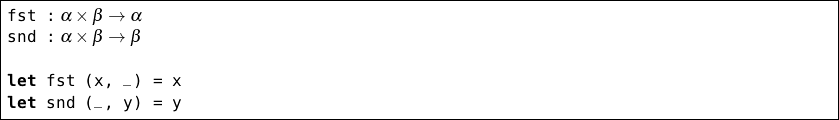Now, we can store a dictionary as a list of pairs: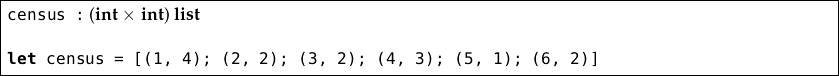Notice the parentheses around int × int in the type. Otherwise, it would be the type of a pair of an integer and an integer list: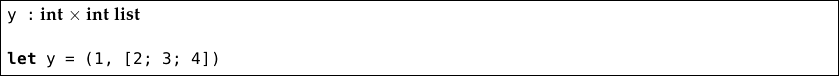What operations might we want on dictionaries? We certainly need to look up a value given a key: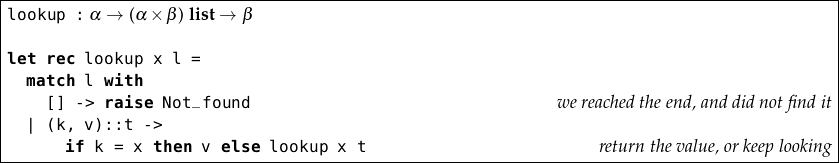For example, `lookup 4 census` evaluates to `3`, whereas `lookup 9 census` raises `Not_found`. Another basic operation is to add an entry (we must replace it if it already exists, to maintain the property that each key appears at most once in a dictionary).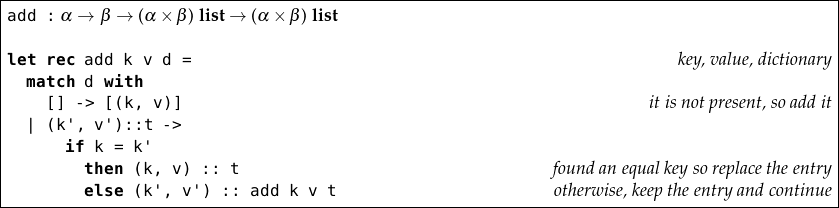For example, `add 6 2 [(4, 5); (6, 3)]` evaluates to `[(4, 5); (6, 2)]` (the value for key `6` is replaced), whereas `add 6 2 [(4, 5); (3, 6)]` evaluates to `[(4, 5); (3, 6); (6, 2)]` (the new entry for key `6` is added). Removing an element is easy: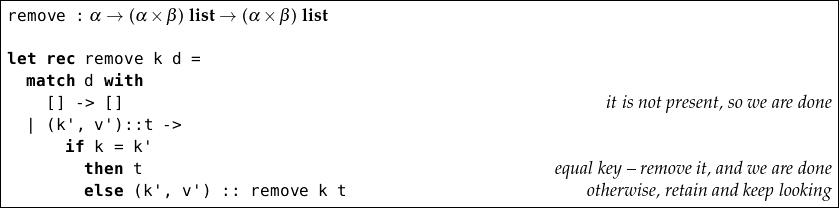The function always succeeds – even if the key was not found. We can use exception handling together with our lookup operation to build a function which checks if a key exists within a dictionary: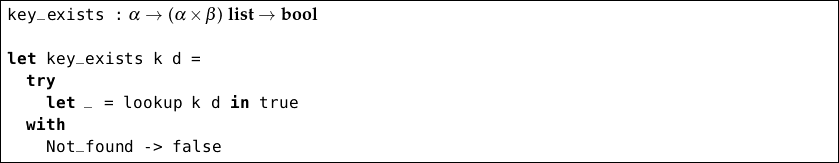If `lookup k d` succeeds, `true` will be returned. If not, an exception will be raised, which `key_exists` will handle itself, and return `false`. Note that we did not give a name to the result of `lookup k l` because we always return `true` if it succeeds.

Pairs are just a particular instance of a more general construct – the tuple. A tuple may contain two or more things. For example, `(1, false, ’a’)` has type int × bool × char.

## Questions

1. Write a function to determine the number of different keys in a dictionary.

2. Define a function `replace` which is like `add`, but raises `Not_found` if the key is not already there.

3. Write a function to build a dictionary from two equal length lists, one containing keys and another containing values. Raise the exception `Invalid_argument` if the lists are not of equal length.

4. Now write the inverse function: given a dictionary, return the pair of two lists – the first containing all the keys, and the second containing all the values.

5. Define a function to turn any list of pairs into a dictionary. If duplicate keys are found, the value associated with the first occurrence of the key should be kept.

6. Write the function `union a b` which forms the union of two dictionaries. The union of two dictionaries is the dictionary containing all the entries in one or other or both. In the case that a key is contained in both dictionaries, the value in the first should be preferred.